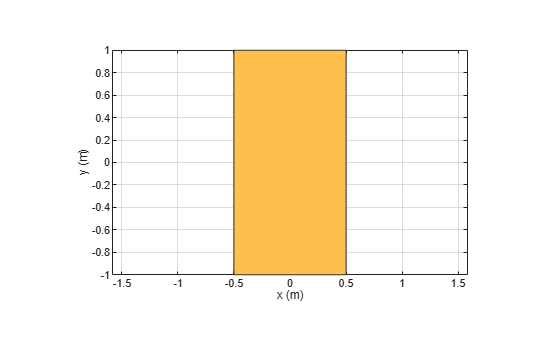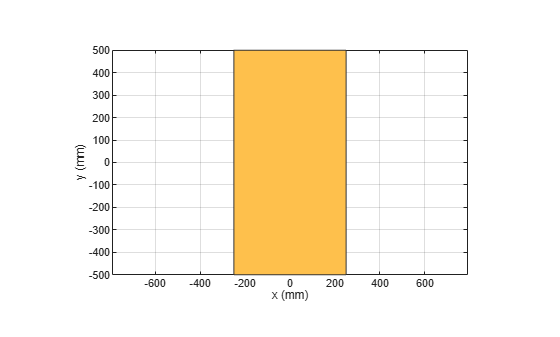scale

Change the size of the shape by a fixed amount

Description

example

c = scale(shape,scaling) scales the shape by a constant factor

Examples

collapse all

Create a rectangular shape.

r  = antenna.Rectangle;
show(r)
axis equalShrink the rectangle by 50%.

scale(r,0.5);Input Arguments

collapse all

Shape created using custom elements and shape objects of Antenna Toolbox, specified as an object.

Example: c = scale(rectangle1,0.5) where rectangle1 is created using antenna.Rectangle object.

Constant factor to change shape size, specified as a scalar.

Data Types: double